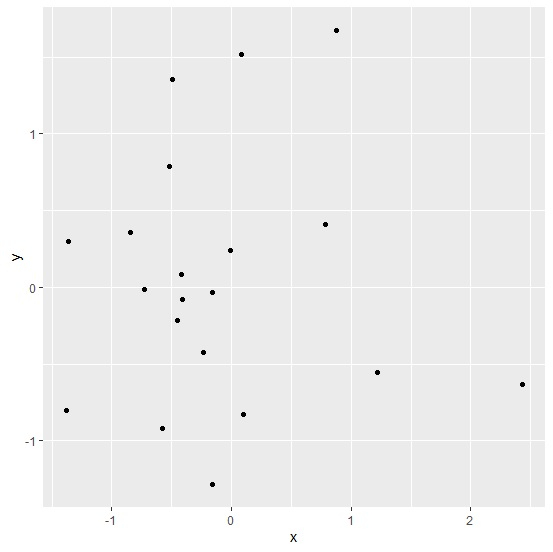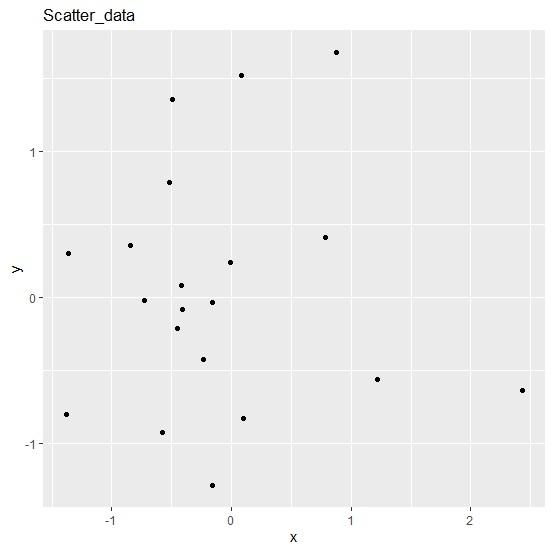# How to display data frame name in ggplot2 graph title in R?n

To display data frame name in ggplot2 graph title, we can use ggtitle function and pass the name of the data frame.

For example, if we have a data frame called df that contains two columns say x and y and we can create a point chart between x and y then the plot with data frame name as title can be created by using the below mentioned command −

ggplot(df,aes(x,y))+geom_point()+ggtitle("df")

## Example

Following snippet creates a sample data frame −

x<-rnorm(20)
y<-rnorm(20)
Scatter_data<-data.frame(x,y)
Scatter_data

## Output

The following dataframe is created −

       x            y
1  -0.845697847   0.35455909
2  -0.407797990  -0.07965950
3  -1.367080923   0.29868478
4  -0.420351817   0.08100959
5  -0.232664470  -0.42197926
6  -0.009006127   0.23762401
7   0.085037204   1.51553702
8   0.878031537   1.67593567
9  -0.727112555  -0.01688995
10 -0.575278542  -0.92132421
11  2.435161686  -0.63178483
12  1.221422580  -0.55697074
13 -0.159321562  -1.28573020
14 -0.448990571  -0.21382377
15  0.101589928  -0.82813014
16 -0.522879574   0.78420506
17 -0.491609573   1.35435264
18 0.788076407    0.41095225
19 -1.381463480  -0.79977881
20 -0.161756452  -0.03442085

To load ggplot2 package and create scatterplot between x and y, add the following code to the above snippet −

library(ggplot2) ggplot(Scatter_data,aes(x,y))+geom_point()


## Output

If you execute all the above given snippets as a single program, it generates the following Output −To create scatterplot between x and y with Scatter_data as plot title add the following code to the above snippet −

## Example

ggplot(Scatter_data,aes(x,y))+geom_point()+ggtitle("Scatter_data")

## Output

If you execute all the above given snippets as a single program, it generates the following Output −